I.5.1abc

6/12/2021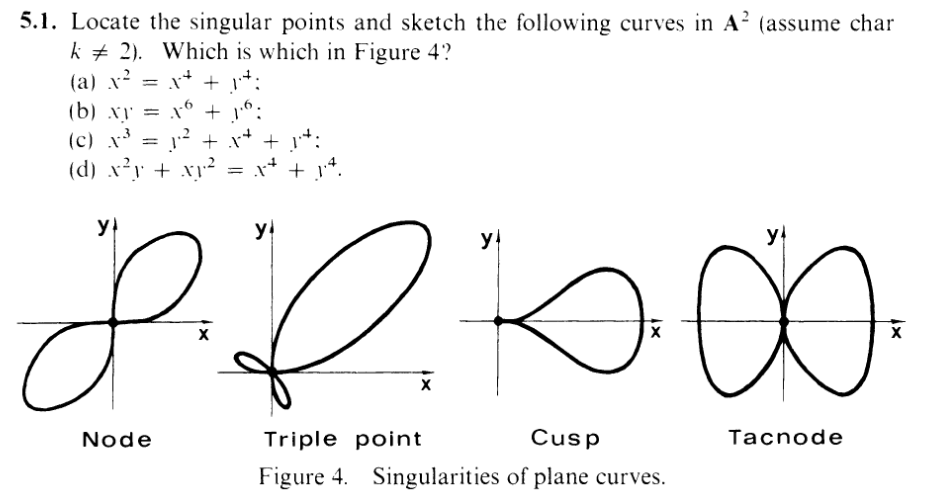Hello, my loves. Welcome to a new section. Let's start by getting the matching out of the way (just graph them on wolframalpha or something):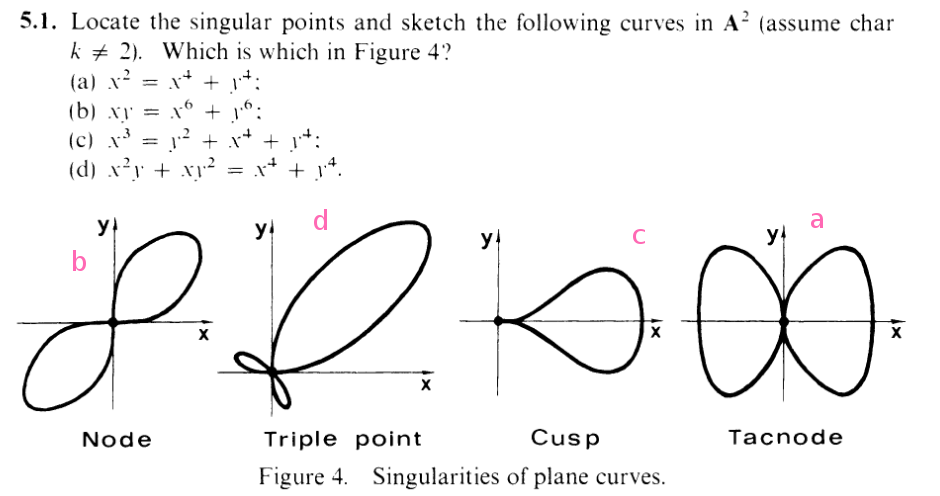The rest has to do with identifying singularities! And this exercise is based on the preliminary definition of (non)singularity: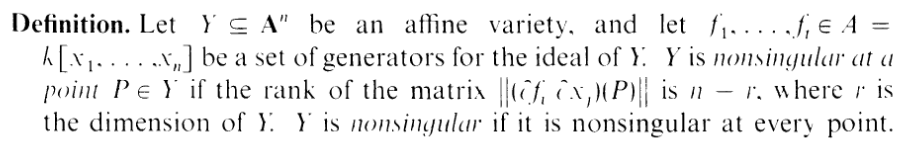Now, as the exercise says, each of the varieties given are curves, which means they each have dimension 1. Hence, for each of these, a point is nonsingular iff the rank of the Jacobian is 2 - 1 = 1. I.e. a point is singular iff the rank of the Jacobian is 0. In particular, the Jacobian in each of these examples is gonna look like

 J =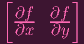(so really, it's just the gradient vector of f) The rank of the matrix is the row span, which is just a single vector, and that is therefore 1 unless both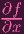and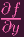are zero. Hence, for each of these problems we are solving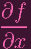= 0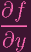= 0

in addition to f = 0, so our solutions actually lie on the curve. Hence this whole exercise is basically solving systems of linear equations (over an algebraically closed field)

"Errr, can't I just look at the graphs and tell from there?" NO. Those graphs are sketches on "real" axes (i.e. non algebraically closed field). Those graphs could be missing some "imaginary"/"complex" singular points, so we do have to go through the algebra (although we do know by looking at the graphs that at least (0, 0) is a singularity for each). See part (c) as an example.

### Part a

Here, f = x4 + y4 - x2. The Jacobian matrix is

 J =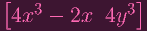Hence, we need

 4x3 - 2x = 0 4y3 = 0

The second equation yields y = 0. And the first equation yields x = 0 or x = ±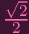. However, (0,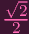) isn't a point on the curve, so the only singular point is (0, 0)

### Part b

Here, f = x6 + y6 - xy. The Jacobian matrix is

 J =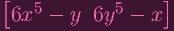Hence, we need

 6x5 - y = 0 6y5 - x = 0

Plugging the second into the first yields

 6(6y5)5 - y = 0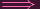66y25 - y = 0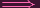y(66y24 - 1) = 0

So y = 0 or y =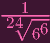Similarly, x = 0 or x =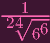Plugging back each into the curve equation, clearly only (0, 0) satisfies it

### Part c

Here, f = x4 + y4 + y2 - x3. So we need

 4x3 - 3x2 = 0 4y3 + 2y = 0

Which factor into

 x2(4x - 3) = 0 2y(y2 + 1) = 0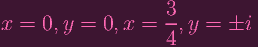By "i" I mean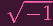, which is defined because we're in an algebraically closed field.

Plugging in all the possibilities back into the curve equation, (0, 0) obviously works and (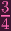, 0) obviously doesn't. Note that

 (-i)4 + (-i)2 = i4 + i2 = (i2)2 + i2 = (-1)2 + (-1) = 1 - 1 = 0

Hence, (0,i) and (0,-i) work and (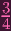,±i) doesn't. So the answers are (0, 0), (0,±i). Note that (0,±i) are points that aren't visible on the "real" graphs on Figure 4.

I cannot fucking figure out how to do part d. The system of equations is

 x4 + y4 - x2y - xy2 = 0 4x3 - 2xy - y2 = 0 4y3 - 2xy - x2 = 0

Reader: If you can solve this system of equations nicely, let me know. Otherwise, I'm skipping it.

(BTW: Some 3s and 6s showed up in this post. I was assuming that the characteristic of k wasn't 3, but if you look back you can see that the case of chark = 3 actually gets us to the answers even more quickly)